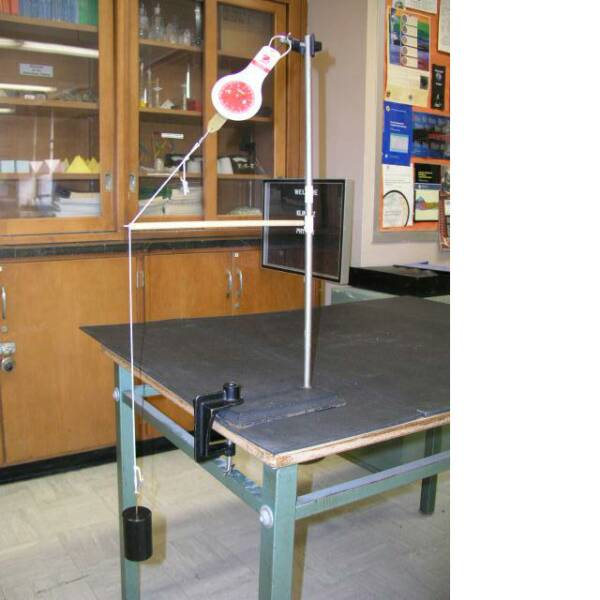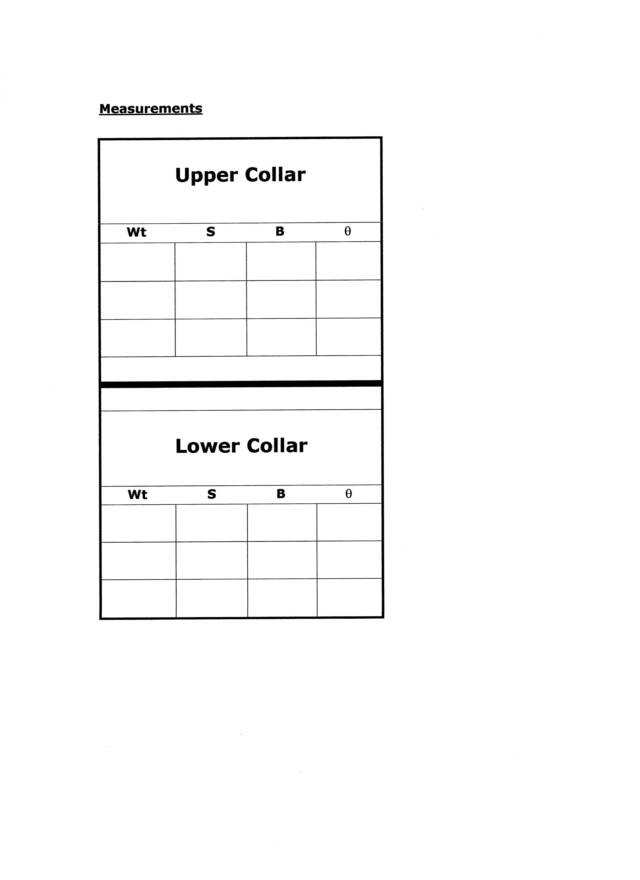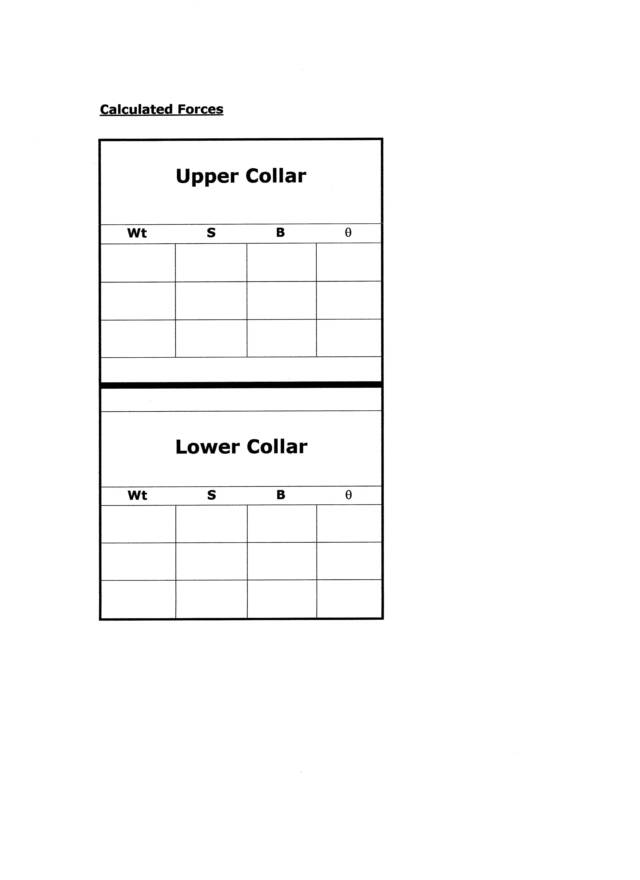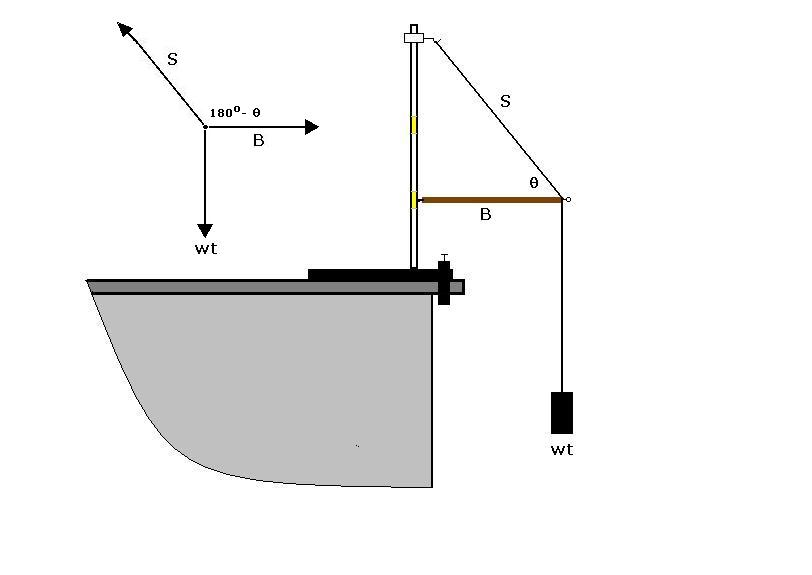Cycle II Physics
John Dewey High School
Mr. Klimetz
Vectors in Statics: The System of Forces in a Boom Crane
Laboratory No. 1
How Do We Resolve a Force Vector into Perpendicular Components?
Objectives. In this exercise you are expected to measure each of the forces acting in a "boom" crane and compare them to the calculated forces as determined from a vector adding diagram. Careful preparation and measurement coupled with proper vector addition can simply illustrate how systems of force vectors combine to yield a zero vector sum in a state of equilibrium.
Materials.
lab stand
prepared wooden dowel "boom" (with nail point and eyelet attached)
two spring scales (calibrated in newtons)
protractor
one hooked 1.0 kg mass
one hooked 0.5 kg mass
set of random hooked masses
0.5-m length of nylon string "guy wire" (looped at each end)
two c-clamps (one large and one small)
Method.1.  Assemble the boom crane apparatus as directed, closely following the assembly diagram placed on the front blackboard and on the picture below.2.  Attach the 0.5-kg mass to the trailing end of the guy wire so that it can be suspended above the floor.3.  Be sure that the contact (nail) end of the boom rests against one of the masking tape "collars" attached to the upright of the lab stand. Additionally, ensure that the boom is oriented orthogonally to the vertical support of the ring stand and that the base of the lab stand is firmly clamped to your table. Use the corner of an index card or square-cut template placed against the upright and the boom to check for proper angle.4.  Measure and/or record the following data:
Suspended Mass (m, kg)
Suspended Weight (wt, N)
String Tension in Guy Wire (S, N)
Angle Between the Guy Wire and the Boom (degrees)
Boom Thrust (B, N)5.  Measuring the boom thrust is accomplished by attaching a force meter to the eyelet of the boom and pulling horizontally outward until the contact between the boom and the upright is broken. The force reading on the meter at the instant of separation equals the boom thrust (B).6.  Repeat steps 2 through 5 after placing the contact end of the boom at the second masking tape collar. Record all data.7.  Replace the 0.5-kg mass with the 1.0-kg mass and repeat steps 2 through 6. Record all data.8.  Suspend the 0.5-kg mass from the bottom of the 1.0-kg mass (for a total of 1.5 kg) and repeat steps 2 through 6.9.  Be sure to measure and record the following for each of the six trials:10. Be mindful to perform any and all conversions as required prior to calculation.
Suspended Mass (m, kg)
Suspended Weight (wt, N)
String Tension in Guy Wire (S, N)
Angle Between the Guy Wire and the Boom (q, degrees)
Boom Thrust (B, N)
Data Analysis and Processing. You must analyze the system of vectors in the boom apparatus both graphically as well as mathematically, in accordance with established vector problem solution methods. As a hint, draw the measured string tension as a vector and then resolve it into horizontal (boom [B]) and vertical (suspended weight [wt]) components. Create acting and adding diagrams for each trial and then provide a mathematical check for comparison.
Questions.
1.Compare the suspended weight (wt) and the vertical component from your diagrams of each trial. Does it seem as if they represent the same force? Explain.
2.Compare the boom thrust (B) and the horizontal component from your diagrams of each trial. Does it seem as if the represent they same force? Explain.
3.Discuss why or why not you believe the forces acting in the boom apparatus can be resolved into horizontal and vertical components. Refer to your graphical and mathematical solutions as proof of the claim that forces can successfully be represented as vectors and combine algebraically and trigonometrically.
4.Define what is meant by the term vector component. Explain why the suspended weight is the vertical vector. Why is it necessary to keep the boom oriented horizontally throughout this exercise?
5.Select your best data and perform a percent error analysis. What does your best data suggest about the forces acting in a boom apparatus and their representation in a vector diagram?
6.How can we demonstrate that the three forces in this system of vectors (B, S, and wt) sum to zero? Why do we say that this functioning apparatus represents an example of static equilibrium?
7.Describe an alternate method of resolving the string tension vector (S) in the guy wire. In other words, imagine that we did not have a spring scale attached to the guy wire throughout the experiment. How could we have solved for the tension in the wire?Tape Collar
Small C-clamp
Spring ScaleGuy Wire
Lab Stand
Large C-clamp
Suspended
Mass
EyeletDowel BoomApparatus and Set-upq90wt
B
S